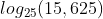## Logarithm

Solve the shown logarithm:Hint
The logarithm of $$x$$ to the Base $$b$$ is defined by
$$log_b(x)=c$$$where $$b^c=x$$ . Hint 2 Factor 25 from 15,625. The logarithm of $$x$$ to the Base $$b$$ is defined by $$log_b(x)=c$$$
where $$b^c=x$$ .

The problem is asking to solve for $$c$$ :
$$36^c=15,625$$$Factor $$25$$ from $$15,625$$ : $$\frac{15,625}{25}=625\rightarrow \frac{625}{25}=25$$$
$$25$$ evenly appears three times in $$15,625$$ . Thus,
$$log_{25}(25^3)=3$$\$
3